## 1、jdk1.7中的HashMap

public class HashMapTest {

public static void main(String[] args) {
}
}

private static AtomicInteger ai = new AtomicInteger();
private static Map<Integer, Integer> map = new HashMap<>();

@Override
public void run() {
while (ai.get() < 1000000) {
map.put(ai.get(), ai.get());
ai.incrementAndGet();
}
}
}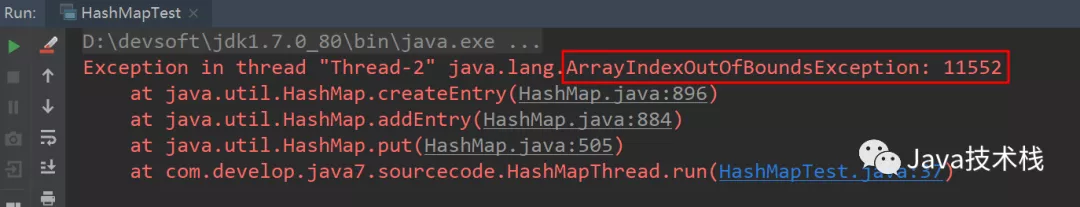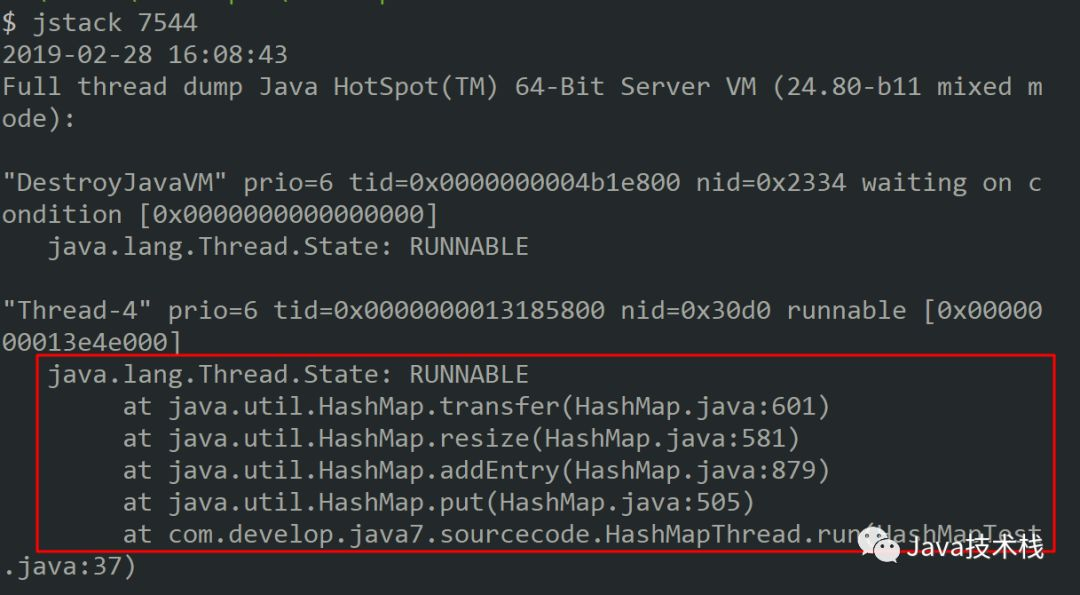void transfer(Entry[] newTable, boolean rehash) {
int newCapacity = newTable.length;
for (Entry<K,V> e : table) {
while(null != e) {
Entry<K,V> next = e.next;
if (rehash) {
e.hash = null == e.key ? 0 : hash(e.key);
}
int i = indexFor(e.hash, newCapacity);
e.next = newTable[i];
newTable[i] = e;
e = next;
}
}
}


### 1.1 扩容造成死循环分析过程

1. hash算法为简单的用key mod链表的大小。

2. 最开始hash表size=2，key=3,7,5，则都在table中。

3. 然后进行resize，使size变成4。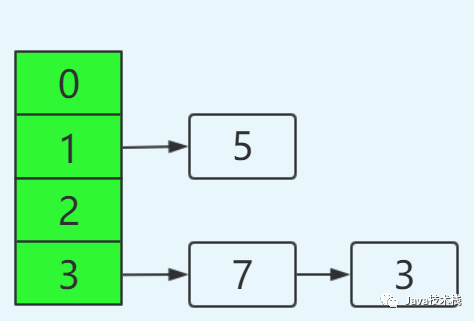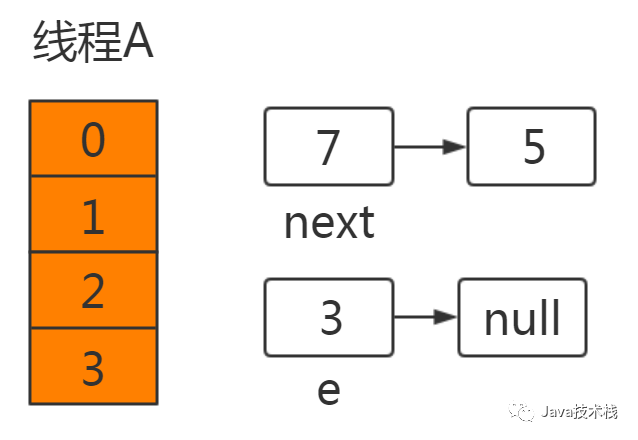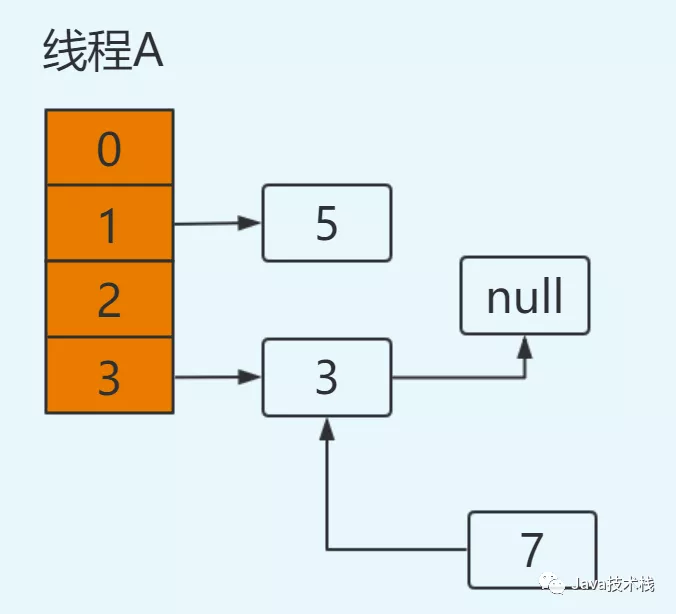e=7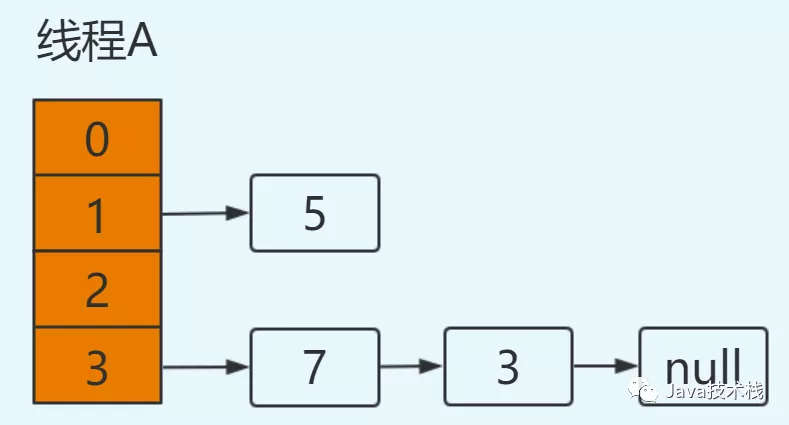e=3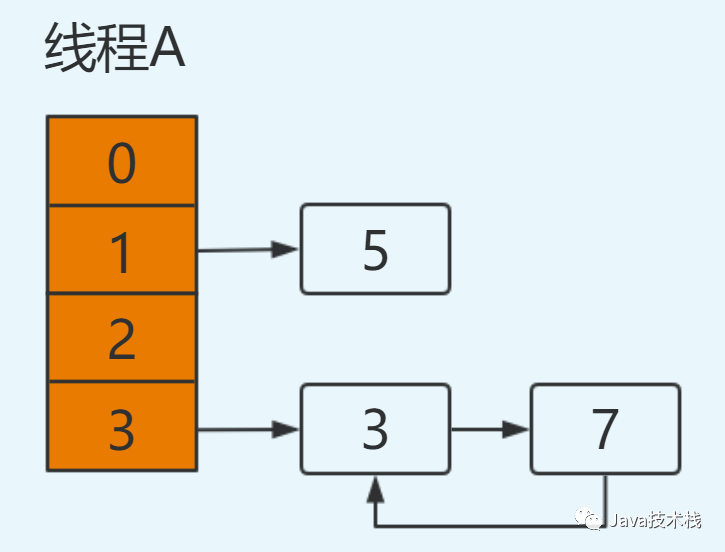### 1.2 扩容造成数据丢失分析过程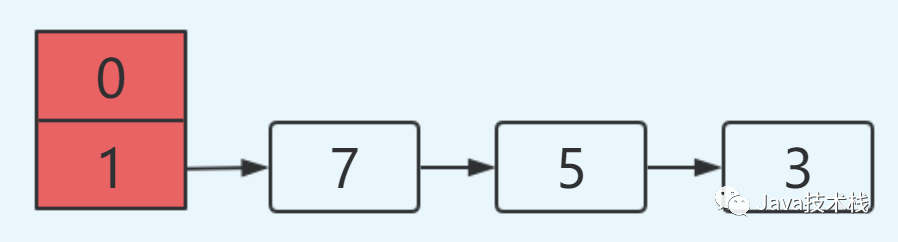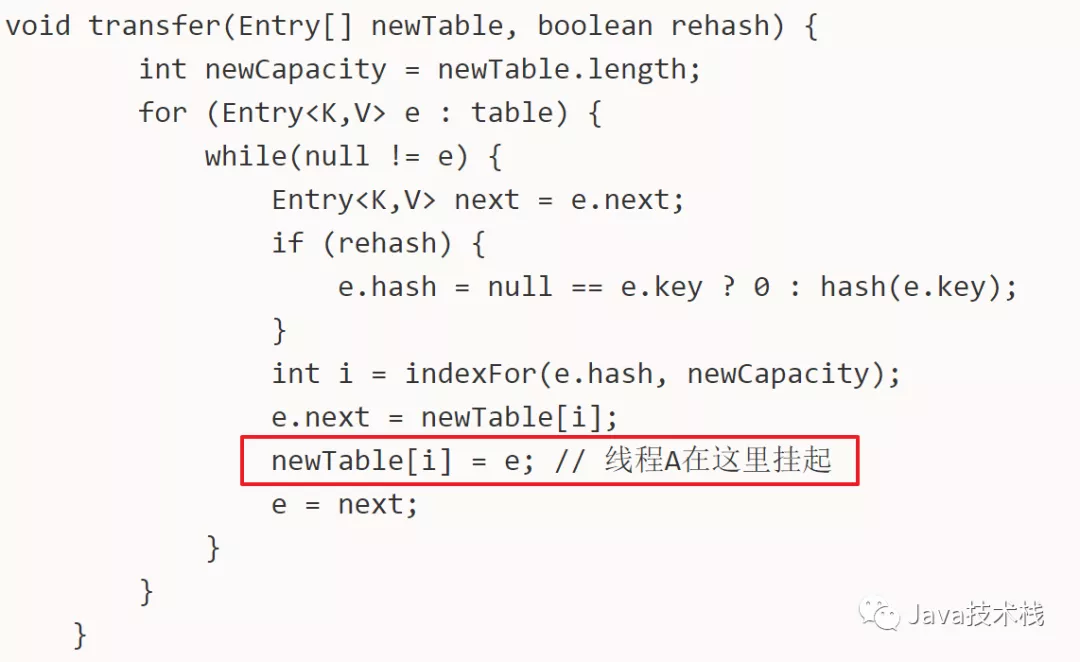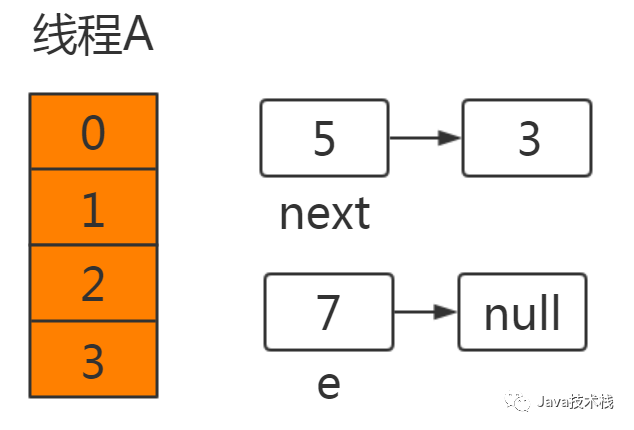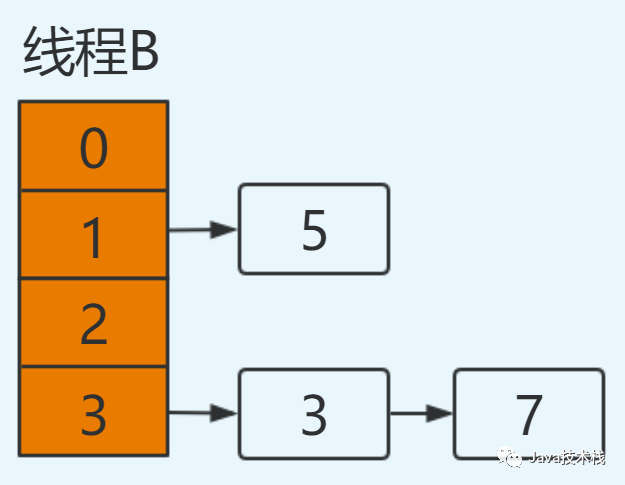e=5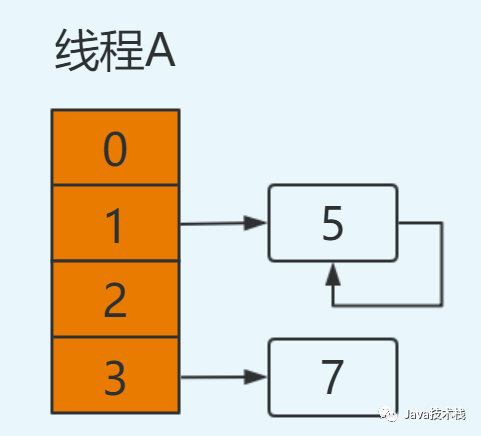## 2、jdk1.8中HashMap

final V putVal(int hash, K key, V value, boolean onlyIfAbsent,
boolean evict) {
Node<K,V>[] tab; Node<K,V> p; int n, i;
if ((tab = table) == null || (n = tab.length) == 0)
n = (tab = resize()).length;
if ((p = tab[i = (n - 1) & hash]) == null) // 如果没有hash碰撞则直接插入元素
tab[i] = newNode(hash, key, value, null);
else {
Node<K,V> e; K k;
if (p.hash == hash &&
((k = p.key) == key || (key != null && key.equals(k))))
e = p;
else if (p instanceof TreeNode)
e = ((TreeNode<K,V>)p).putTreeVal(this, tab, hash, key, value);
else {
for (int binCount = 0; ; ++binCount) {
if ((e = p.next) == null) {
p.next = newNode(hash, key, value, null);
if (binCount >= TREEIFY_THRESHOLD - 1) // -1 for 1st
treeifyBin(tab, hash);
break;
}
if (e.hash == hash &&
((k = e.key) == key || (key != null && key.equals(k))))
break;
p = e;
}
}
if (e != null) { // existing mapping for key
V oldValue = e.value;
if (!onlyIfAbsent || oldValue == null)
e.value = value;
afterNodeAccess(e);
return oldValue;
}
}
++modCount;
if (++size > threshold)
resize();
afterNodeInsertion(evict);
return null;
}


## 总结

1. 在jdk1.7中，在多线程环境下，扩容时会造成环形链或数据丢失。

2. 在jdk1.8中，在多线程环境下，会发生数据覆盖的情况。